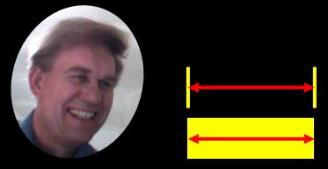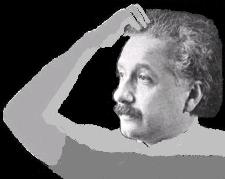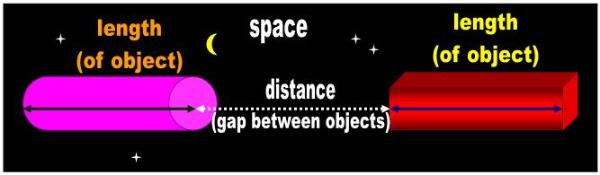Adapted for the Internet from:Why God Doesn't Exist
 Length and distanceare not synonyms for the purposes of Science

1.0   The mathematicians say that length and distance are synonyms

Einstein tried his hand at a definition of distance and failed miserably. Like all mathematicians, he confused distance with
length:

“ Distance denotes a rigid body on which two material points (marks) have been
specified.”  

[NOT! ]

[No Al. You are not defining distance. You are defining length! I recommend
holding Al back another year in kindergarten until he learns the fundamentals
of Physics.]

If Einstein is going to invoke a body, meaning a physical object, he is not talking about distance.I have no idea what you're talking about, Bill. In relativity, length and distance are synonyms.2.0   Length is to matter what distance is to space

Matter is said to consist mainly of empty space:

“ A cloud of tiny negatively-charged electrons envelopes the nucleus,
but at a relatively large distance, so that much of the volume of an
atom is empty space.” 

“ Ordinary matter, or the stuff we and everything around us is made of,
consists largely of empty space. Even a rock is mostly empty space.” 

In spite of this, the mathematicians do not subtract the 'space bubbles' from the length of the table. They treat the table as if
it were a continuous object: made of a single chunk of matter. Length is a property restricted to physical objects and is
conceptually 'made' of a single piece. We can chop the ruler in half, but not its length. We don't slice concepts with a chain-
saw. We cut physical objects, you know, like trees and chairs and heads, with a saw. For the purposes of Physics, length is
indivisible.

Conversely, distance is a property we assign to space. Therefore, if a physical object is conceptually continuous and space
is what interrupts this continuity, it follows that space is what is really discontinuous. It is space which enables objects to be
discrete. Without space, the entire Universe would be a single block of infinite matter! Ironically, the mathematicians have
concluded exactly the opposite:

“ In general relativity, space-time is assumed to be smooth and continuous- and
not just in the mathematical sense. 

However, you should not lose sight of the context. In Science, we use adjectives (such as discontinuous) to modify objects.
We use the opposites of these adjectives to characterize space       (e.g., discontinuous, transparent, intangible). This is
simply a convention having roots in our peculiar vernacular. We could just as well argue that adjectives should be
circumscribed to objects and not be applicable to space. Certainly, space cannot be white, big, flat, or three-dimensional.

So what does the word distance mean for the purposes of Physics? How can we use this word consistently in a dissertation
(i.e., scientifically)?

If you look the word up in the dictionary or ask anyone on the street, you get pretty much the intuitive ‘physical’ definition of
the word distance:

“ the extent or amount of space between two things, points, lines, etc.; the state
or fact of being apart in space, as of one thing from another”  

This definition contains the essence of what distance means in Science. For the purposes of Physics, length is to matter
what distance is to space. Distance refers to the absence of matter: to the void between two objects (Fig. 1). Length is what
ONE object has; distance is what it doesn't.  Distance is what stands between TWO objects! In Physics, we talk about the
length of the ruler and the distance between two telephone poles.

length: Continuous matter lying between two surfaces.

distance: The space between two surfaces. A discontinuity in matter.

Therefore, for the purposes of Physics length is not the same as distance and this should be pointed out to the idiots of
Mathematics. They need to be re-educated if they are ever to understand anything about Physics. It can no longer be
tolerated for graduates to make such basic errors.

In short, distance refers to the separation between TWO objects. This is what a sane human being understands by
distance. Distance insulates objects because it is a subset of space, yet distance and space are not identical or
synonymous. Whereas space is the ubiquitous background that contours matter, distance is the particular space that
separates any TWO surfaces. Distance separates two particular faces. In contrast, space contours each object all around.
Unlike space, distance embodies direction and is conceptually the extension from one side of an object to another. If we
use a single point lying on an object as a reference, the point is effectively assumed to be structural (i.e., a dot) and to
possess a face. The mathematicians may argue that they can use a point-particle as the center of mass of an object. They
may not use the point-particle as a physical entity that is separated by distance from another one. There is no such notion
as distance between locations. In Physics, we may only talk about distance between objects. Distance is unimaginable
between zero-dimensional (0D), face-less concepts such as point particles and locations.

Distance also differs from space in that it is an artificial concept. Distance is a notion we conceived. Space was there even
before God was around.
 distance
 lengthYou see, Bill. In Mathematics we determine the length from front to back by measuring the distance the sword travels.
 That's great, Steve, but wouldn't it have been easier on Al if you had  measured the length of his neck with this cloth instead?We have no use for a stick in Mathematics, Bill! We measure length and distance by counting the footprints we make with our bare feet.
 Fig. 1   Length vs. distance
 The word 'length' refers to the continuous matter lying between two surfaces of ONE object. The word 'distance' refers to a region  devoid of matter: to the space that separates TWO surfaces. Length is to matter what distance is to space. Both length and distance are conceptually static.The fanatics of the religion of Mathematical Physics today make the same mistake as their mentor. They claim that the words
length and distance are synonymous:

“ In the physical sciences and engineering, the word ‘length’ is typically
used synonymously with ‘distance’ 

On the one hand, the mathematicians define length as the ‘distance’ between two points:

" Length, of a straight line segment, the distance between its end points" 

" length: the longest extent of anything as measured from end to end...The linear
distance between two points. " 

" Length is defined as the straight-line distance between two points along
an object." 

On the other, they define distance in terms of 'length':

" The distance between two points is the length of a straight line between them.” 

" distance: The length of the line segment joining two points. A straight line
segment is the shortest distance between two points." 

" The one-dimensional parallelepiped is called distance" (p. 20) 

Of course, if relativists rely on circular definitions and synonyms, they will never understand Physics. If in 4000 years, our
absent-minded mathematicians have not figured out that there is a non-trivial, qualitative difference between length and
distance, what can you expect from the physical interpretations these morons derive from equations that deal with these
parameters?

Therefore, before we say another word, we need to show the mathematical establishment the error of their ways. We
must establish a clear difference between length and distance. We will otherwise be unable to discuss issues such as
length contraction in a rational manner.________________________________________________________________________________________